Learn how »

# Mathematica for University Research

In this Wolfram Training course, learn why Mathematica is used for academic research with a look at its programming language, support for parallel computing, and multiple publishing and deployment options.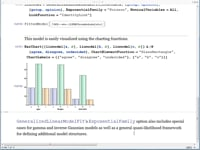Darren Glosemeyer Model fitting and analysis topics covered in this Wolfram Training on-demand course include Mathematica's framework and functionality for linear and nonlinear regression; logit, probit, and other generalized linear models; and ...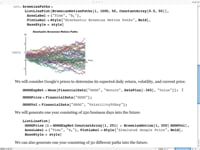Samuel ChenFor financial analysts, it takes a considerable amount of effort to gather and validate data, perform statistical or pricing analysis, and generate reports that are easily understood by clients or ...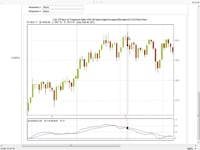Michael Kelly The data analysis, modeling, and visualization aspects of Wolfram Finance Platform are explored in this Wolfram Training on-demand course. You'll also learn how to create interactive tools for analyzing and ...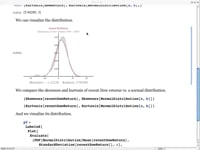Samuel ChenStatistical methods provide a powerful tool for analyzing and drawing conclusions from financial data. This Wolfram Training on-demand screencast showcases the application of probability, distribution, and statistical analysis to financial ...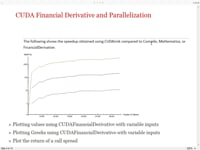Michael Kelly Learn to use Mathematica's financial derivatives functions, including CUDA, the use of SymbolicC for GPU creation, stochastic differential equations, and more in this Wolfram Training on-demand course.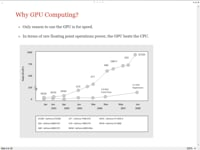Abdul Dakkak This Wolfram Training course provides an introduction to the new GPU functionality in Mathematica 8 and shows examples of how to deploy your solution and scale across GPUs and machines.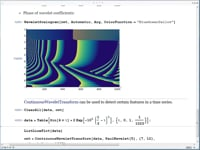Paritosh Mokhasi Wavelet analysis has been applied to a broad range of fields. In this Wolfram Training on-demand course, examples are presented from applications including financial time series, edge detection and denoising ...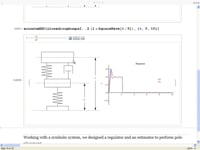Bob Sandheinrich This Wolfram Training on-demand course gives a step-by-step approach to working with control systems and state space applications using Mathematica. Each example begins with a problem definition and works toward a solution and ...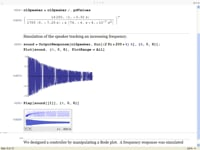Bob Sandheinrich This Wolfram Training on-demand course gives a step-by-step approach to working with control systems and frequency domain applications using Mathematica. Each example begins with a problem definition and works toward a solution and ...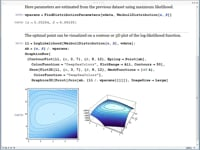Darren Glosemeyer This Wolfram Training on-demand course gives a comprehensive look at statistical distributions in Mathematica, including functions for constructing or deriving new distributions, automated estimation of distribution parameters, and goodness-of-fit testing ...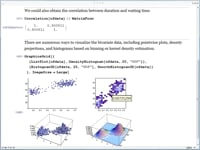Darren Glosemeyer Mathematica provides numerous functions for describing and visualizing properties of data. This Wolfram Training course covers shape statistics, measures for multivariate data, charts, and plots.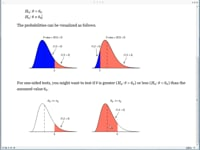Darren Glosemeyer Mathematica's framework for hypothesis testing includes parametric and nonparametric tests of location, variance, or scale. Additional topics covered in this Wolfram Training on-demand course include functions for common named tests, ...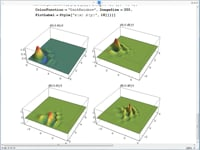Paritosh Mokhasi This Wolfram Training on-demand course covers continuous, discrete, and stationary wavelet transforms, as well as wavelet algorithms and visualizations.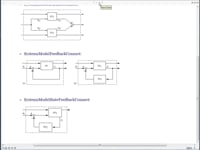Mili VishwakarmaThis Wolfram Training on-demand course introduces the concepts and Mathematica functions used to model a dynamic system. Topics include the construction and manipulation of state space and transfer function models, system properties, ...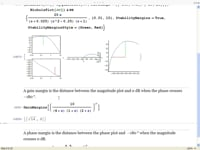Bob SandheinrichThis Wolfram Training on-demand course shows how to work with the control systems objects created in Mathematica. Topics include frequency domain analysis, state space analysis, and state space design.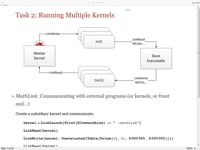Yu-Sung ChangThis scenario-driven Wolfram Training course covers topics applicable to enterprise-wide projects: large database access, dynamic visualization, and deploying a web interface with CDF technology.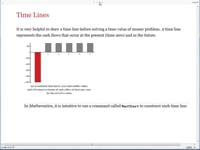Samuel ChenWith customized financial examples such as discounted cash flows and mortgage valuation, this Wolfram Training course introduces the mathematics of time value and the built-in Mathematica functions that make calculations ...Yu-Sung ChangMathematica provides a powerful graphics language for general use and highly sophisticated visualization. This Wolfram Training course covers topics such as lighting control, camera setup, texture, and more.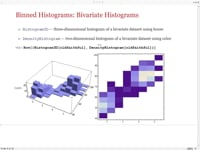Brett ChampionThis Wolfram Training on-demand course provides an overview of some of the new statistical visualization functionality in Mathematica 8. Topics include visual inspection of the shape of data and comparisons to ...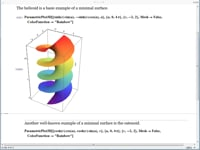Devendra KapadiaThis Wolfram Training on-demand course provides applications of calculus functions in Mathematica and a discussion of the internal methods used for solving problems.